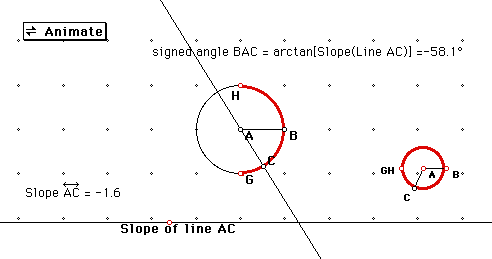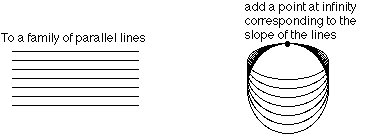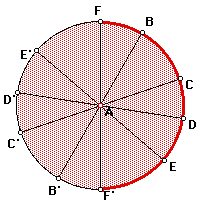# Projective geometry and slopes

If you have the package Geometer's Sketchpad installed on your computer, you can download and run an interactive sketch. First, configure your web browser to open Geometer's Sketchpad as a helper application; here are instructions. You can download a demo copy of Geometer's Sketchpad (Macintosh or Windows) from Key Curriculum Press.

Here is the interactive sketch. Otherwise you can look at this sequence of pictures.Picture 2.
Picture 3.
Picture 4.
Picture 5.

In the sketch, line AC rotates around the origin, A. The rotation is controlled by point C on the unit circle. At the bottom of the sketch, there is a number line on which is plotted the slope of line AC. Double-click the Animate button and watch (or imagine) how the slope of AC changes as C moves around the circle.

Things you might have noticed:

• As the line rotates counterclockwise, its slope increases. At the instant it becomes vertical, its slope is undefined. [Note about computers and roundoff errors.] As the line moves off the vertical slope position, its slope is in the large negative values, which increase towards 0 as the line continues to rotate.
• As C moves along the right semicircle (in red), every real number, positive and negative, occurs as a slope. As C moves along the left semicircle, these values are repeated.
• If you watch the point representing the slope move along the number line, you will see it disappear off the right edge and reappear on the left.
It makes sense (at least in the context of projective geometry, explanation below) to fill in the missing slope value for vertical lines by connecting the right and left "ends" of the number line of slopes with a single "point at infinity". This point represents a vertical line, which has infinite slope: the closer a line gets to vertical the bigger its slope gets (in absolute value).

If connecting the ends of an infinitely long line makes you really nervous, use the right red semicircle instead. This represents all slopes, negative and positive, and also represents the corresponding angles that the line makes with the x axis: from -90 degrees to 90 degrees. This gives a one-to-one (and continuous, for those who know calculus) correspondence between the points of the number line and the points of the semicircle, excluding its endpoints at -90 and 90. Now bend this semicircle until the two missing endpoints come together and fill in the gap with a point at infinity. You get a circle, or at least a loop. Each point of this circle corresponds to a distinct slope, including vertical lines. As point C goes around the original circle once, the corresponding point goes around the smaller circle twice.

## Projective geometry

Projective (plane) geometry is a type of (non-Euclidean) geometry that is an extension of Euclidean plane geometry. In addition to all the points in the plane, there are extra "points at infinity"; the idea is similar to what happened in the paragraphs above. Consider a line through the origin in the plane, such as line AC above. Just as a point at infinity was added to the number line, add a point at infinity to this line. In fact, add the point on the number line representing its slope. (Think of this number line as being somewhere else, not in the same plane as line AC.) For each line with a different slope, add its own point at infinity from the line of slopes. For the vertical lines, add the point at infinity on the line of slopes that we constructed before.

Interactive sketch.Now we have a Euclidean plane, a new line of points at infinity, and a point at infinity for the line at infinity. (All the points at infinity form a projective line , which has the shape of a loop.) We also define some new "incidence" relations: what points are on what lines, and what lines intersect at what points.

A point at infinity is on every line that has the slope it corresponds to.Every point at infinity is also on the new line at infinity. So now two lines always intersect: parallel lines intersect at the point at infinity corresponding to their common slope. The line at infinity intersects any regular line at the point at infinity corresponding to the regular line's slope.

The Euclidean plane is an infinite flat surface. What does the projective plane look like, once all these new strange points are added? Here are some pictures.

More explanations of the pictures:
The projective plane doesn't go off to infinity; all the infinite lines have been turned into finite loops.

To see how to get these strange shapes by adding new points to the plane, first shrink the entire plane onto a disk that doesn't include its boundary. You can think of this disk as the interior of the circle that point C moves on in the first sketch. A line through the origin in the plane now corresponds to a diameter of this disk.The two missing endpoints, for example, C and C', of the diameter correspond to the "ends" of the original line. Connect the ends of the diameter with a point at infinity corresponding to the slope of the diameter. Now it's a loop, not a line. Do this to every diameter. Note that you have to do it only for half the circumference of the disk, since the other half is taken care of by the other endpoint of each diameter.

If you try to do this procedure with a piece of cloth, you will see that you soon get a snarly mess. The disk has to cross through itself to finish all the connecting. The pictures you saw are what you would get if you could actually do this.

To Susan Addington's home page at the Geometry Center